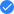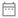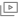Back to Plus

# Course on Engineering Mathematics & Numerical Analysis

Prakhar Shrivastava16k followers

In this course, Prakhar will discuss core concepts of mathematics from the exam perspective along with numerical solving ability. USP is its practical life examples and connection between subject and student. This course will be beneficial for aspirants preparing for ESE exams. This course will be taught in English and notes will be provided in English.
Course schedule
Aug
08
Introduction & Analysis of Engineering Mathematics
Lesson 17:30 AM
Aug
09
Matrix Introduction
Lesson 27:30 AM
Aug
10
Ranks and System of linear equation
Lesson 37:30 AM
Aug
11
Doubt Clearing Session
Lesson 47:30 AM
Aug
12
Eigen Values and Vectors
Lesson 57:30 AM
Aug
13
Introduction to Complex Numbers
Lesson 67:30 AM
Aug
14
Analytic equation & Cauchy Reimen Equation
Lesson 77:30 AM
Aug
15
Doubt Clearing Session
Lesson 87:30 AM
Aug
16
Integral Theorem and Residues
Lesson 97:30 AM
Aug
17
Taylor & Laurent Series
Lesson 107:30 AM
Aug
17
Weekly Quiz 1
Lesson 1110:30 AM
Aug
17
Doubt Clearing Session
Lesson 1210:30 AM
Aug
18
Introduction to Probability
Lesson 137:30 AM
Aug
18
DE - Introduction to Differential Equations
Lesson 1410:30 AM
Aug
19
Doubt Clearing Session
Lesson 157:30 AM
Aug
19
DE - Solution of Differential Equation
Lesson 1610:30 AM
Aug
20
Probability - Operations and Theorem in Probability
Lesson 177:30 AM
Aug
20
DE - Higher order Differential equation
Lesson 1810:30 AM
Aug
21
Statistic Introduction - Mean, Median& Mode
Lesson 197:30 AM
Aug
21
Laplace Equation
Lesson 2010:30 AM
Aug
22
DE - Solution to Heat, Wave and Other Equations
Lesson 213:30 AM
Aug
22
Probability Distributions - Part 1
Lesson 227:30 AM
Aug
23
Doubt Clearing Session
Lesson 237:30 AM
Aug
24
Weekly Quiz 2
Lesson 2410:30 AM
Aug
31
Doubt Clearing Session
Lesson 257:30 AM
Aug
31
Weekly Quiz 3
Lesson 2610:30 AM
Sep
02
Calculus - Differentiation & THEOREMS
Lesson 275:00 AM
Sep
02
Calculus - Limits and Continuity
Lesson 287:30 AM
Sep
03
Calculus - Differentiation
Lesson 297:30 AM
Sep
03
Weekly Quiz 4
Lesson 3010:30 AM
Sep
04
Doubt Clearing Session
Lesson 317:30 AM
Sep
05
Calculus - Application of derivative - 1
Lesson 327:30 AM
Sep
06
Calculus - Application of derivative - 2
Lesson 337:30 AM
Sep
07
Calculus - Partial & Total Derivative
Lesson 347:30 AM
Sep
08
Doubt Clearing Session
Lesson 357:30 AM
Sep
09
Calculus - Definite and Improper Integral
Lesson 367:30 AM
Sep
10
Calculus - Double and Triple Integrals
Lesson 377:30 AM
Sep
11
Weekly Quiz 5
Lesson 3810:30 AM
Sep
12
Doubt Clearing Session
Lesson 397:30 AM
Sep
13
Calculus - Series
Lesson 407:30 AM
Sep
14
Vectors - Introduction
Lesson 417:30 AM
Sep
15
Vectors - Gradient, Divergence and Curl
Lesson 427:30 AM
Sep
16
Doubt Clearing Session
Lesson 437:30 AM
Sep
17
Vectors - Identities and Questions
Lesson 447:30 AM
Sep
18
Vectors - Line, Surface and Volume Integral
Lesson 457:30 AM
Sep
18
Weekly Quiz 6
Lesson 4610:30 AM
Sep
19
Vectors - Green's, Gauss and Stokes theorem
Lesson 477:30 AM
Sep
20
Doubt Clearing Session
Lesson 487:30 AM
Sep
21
Numerical Method - Introduction
Lesson 497:30 AM
Sep
22
Numerical Method - Linear and non linear algebraic equation
Lesson 507:30 AM
Sep
23
Numerical Method - Integration - Trapezoidal etc.
Lesson 517:30 AM
Sep
24
Doubt Clearing Session
Lesson 527:30 AM
Sep
24
Weekly Quiz 7
Lesson 5310:30 AM
Sep
25
Numerical Method - Differential Equation Solution
Lesson 547:30 AM
Sep
26
Revision 1
Lesson 557:30 AM
Sep
27
Revision 2
Lesson 567:30 AM
Sep
28
Mega Quiz
Lesson 5710:30 AMCourse will be taught in English8th August to 28th September 201949 lessons, 8 quizzes